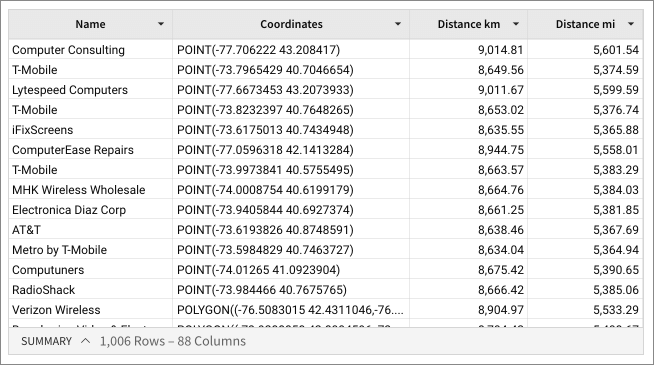# Distance

The Distance function calculates the minimum distance between two geographies, in specified units.

You can use the Distance function on Snowflake and BigQuery connections.

Distance is one of Sigma's Geography functions.

## Syntax

`Distance(units, geog1, geog2)`

The function arguments are:

units
Required
The unit of measurement for the distance
Valid values are `"kilometers"`, `"meters"`, and `"miles"`.
geog1
Required
First geography of the pair between which we calculate the distance
Must be in valid Geography format.
To work from known latitude and longitude values, use the MakePoint or MakeLine functions.
geog2
Required
Second geography of the pair between which we calculate the distance
Must be in valid Geography format.
To work from known latitude and longitude values, use the MakePoint or MakeLine functions.

## Examples

`Distance("kilometers", [Coordinates], MakePoint(0, 0))Distance("miles", [Coordinates], MakePoint(0, 0))`

The Distance function returns the following values for the Coordinates column, when specifying distance in either kilometers or miles from coordinates (0, 0):